# TECH SPOT: SAMPLE CRE QUESTIONS (Part 22)1. Battery life has been measured as normally distributed with mean equal to 150 hours and variance of 400 hours. Find the B10 life.
A.124.4 hrs

B. 175.6 hrs

C.117 hrs

D. 362. hrs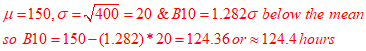2. How should a Reliability Engineer react when a corrective action was rejected by management?

A. Insist the corrective action must be implemented,

B. Implement corrective action by oneself,

C. Agree with management and document the findings,

D. Agree with management and discard the findings

3. Among the various reliability tests that are performed on products at various stages of development, which of the following tests are most useful in determining the minimum mean time to failure requirements:

1) Reliability growth testing,

2) Environmental stress screening,

3) Reliability qualification testing,

4) Acceptance testing

A. 1&2,

B. 2&3,

C. 3&4,

D. 4&1

4. In a sequential reliability test, if the outcome of the test is pass or fail for each individual item, and if the producer’s risk is α and the consumer’s risk is β, the decision to continue testing Z as a function of α and β is:

𝐴.(1−𝛽/𝛼)<𝑍<(𝛽/1−𝛼),

𝐵.(𝛽/1−𝛽)<𝑍<(𝛼/1−𝛼)

𝐶.(𝛼/𝛽)<𝑍<(1−𝛼)/(1−𝛽),

𝐷.(𝛽/1−𝛼)<𝑍<(1−𝛽/𝛼)

5. If the probability density function under accelerated conditions is f(t) with ε being the acceleration factor, then the probability density function f0 under normal operating conditions is:

A. f0 = (ε)f(t/ ε),

B. f0 = (1/ ε)f(t/ ε),

C. f0 = (1/ ε)f(ε / t),

D. f0 = (1/ ε)f(t/ ε) 2

6. The average U.S. dollar/Euro exchange rate from a sample of 36 monthly observations is \$1.00/Euro. The population variance is 0.49. What is the 95% confidence interval for the mean U.S. dollar/Euro exchange rate?
A. \$0.8657 to \$1.1343,

B. \$0.5100 to \$1.4900,

C. \$0.763 to \$1.236,

D. \$0.8075 to \$1.1925,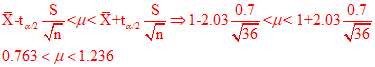7. Considering the confidence level formula, if sample size increases
by 4, the width of the interval would change by which value?
A. Decreases by ½,

B Decreases by ¼,

C. Increases by 2,

D. Increases by 4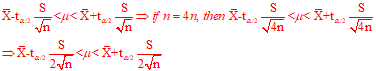8. A system has three subsystems with a reliability of R. System success requires that at least two of the subsystems operate. The system reliability can be calculated as:
A. 3R3-2R2,

B. 3R2-2R3

C. 3R2-2R3

D. 2R2-3R3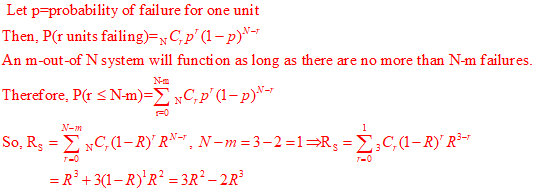9. If the system reliability of this system is 0.93,     what is the reliability value for component A?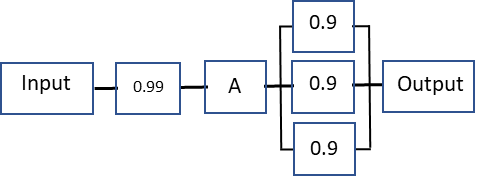A. 0.94,

B. 0.93

C. 1.29

D. 0.92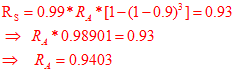10. An electronic system reliability block diagram shows as the following:What is the system Failure Rate in FITS?
NOTE: The sub‐system failure rate is in FITs. FITs = Failures per billion operating hours
A. 1500,

B. 3000,

C. 4000,

D. 5000

Ans:
Subsystem #1 FR in FITS=1500/1E9
Subsystem #2 FR in FITS=1500/1E9
Subsystem #3 FR in FITS=2000/1E9
So, System FR= 1500/1E9+ 1500/1E9 + 2000/1E9= 5000/1E9

Scroll to Top